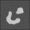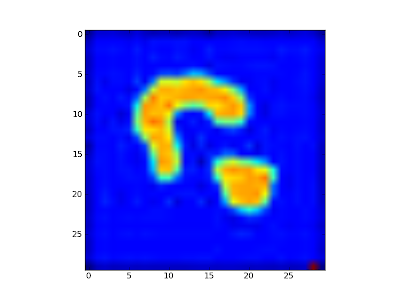## Saturday, July 23, 2011

### Clustering, Image Segmentation, Eigenvectors and Python

Here is example code for eigenvector based segmentation in Python. For more details, see code here.
```import matplotlib.pyplot as plt
import numpy as np

n = Img.shape
Img2 = Img.flatten(order='C')
nn = Img2.shape

A = np.zeros((nn,nn))

for i in range(nn):
for j in range(nn):
A[i,j]=np.exp(-((Img2[i]-Img2[j])**2))

V,D = np.linalg.eig(A)
V = np.real(V)
a = np.real(D)
print a

threshold = 0 # filter
a = np.reshape(a, (n,n))
Img[a<threshold] = 255
plt.imshow(Img)
plt.show()```